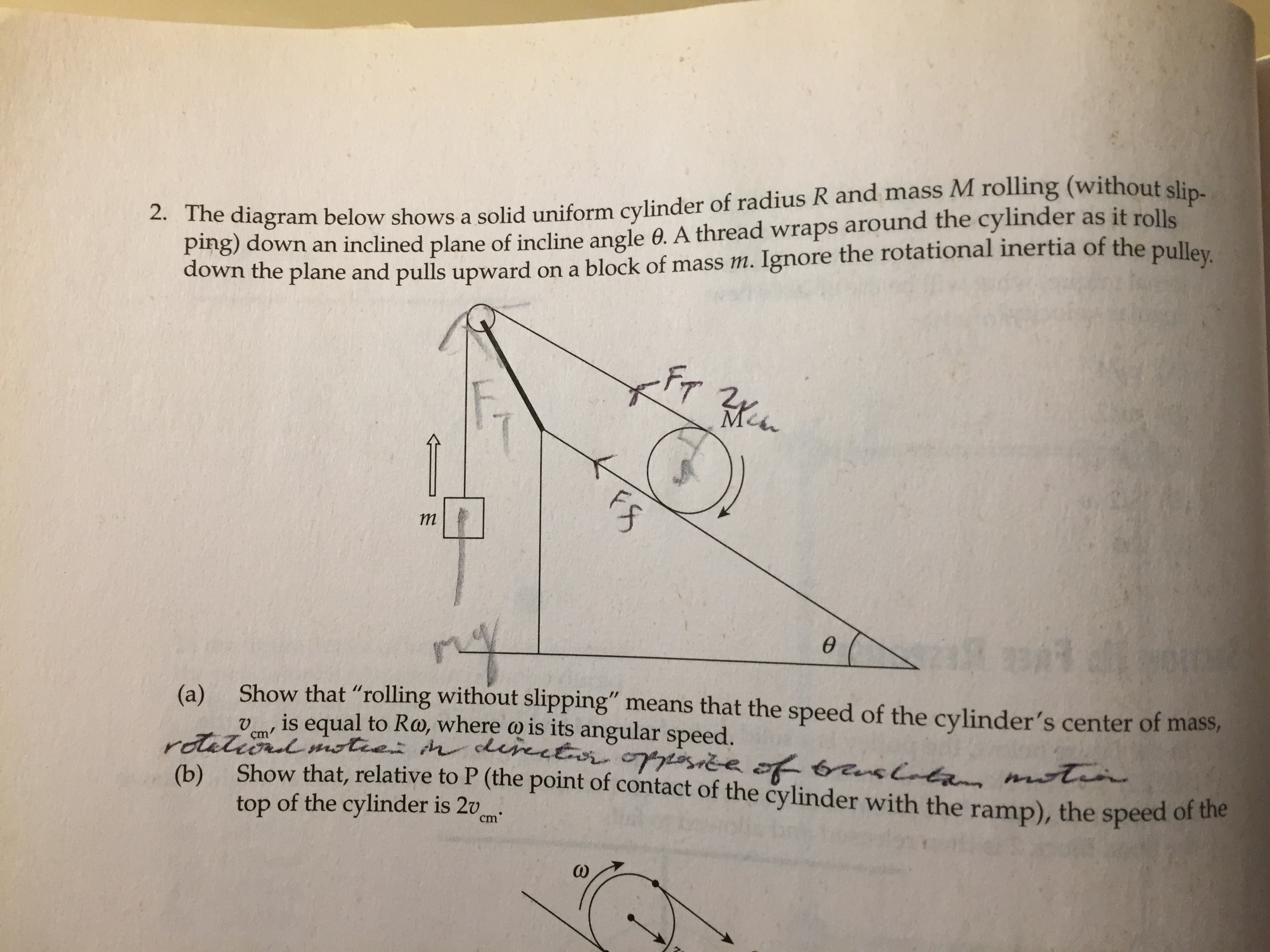# A mechanics problem

Today, I solved one from the Cracking the AP Physics C Exam 2008 Edition, which I had bought in high school. It isQuestions (c), (d), and (e) are not included. They are to determine the relationship between the magnitude of the acceleration of the block and the linear acceleration of the cylinder, to determine the acceleration of the cylinder, and to determine the acceleration of the block respectively. It is obvious that from (b) that the magnitude of the acceleration of the block is twice the linear acceleration of the cylinder, and the answer to (e) can be derived from that of (d) via this. As for (d), I first solved it with a free body diagram and Newton’s laws. Then, my ultra gifted friend Brian Bi suggested using the Euler-Lagrange equations, which simplifies things greatly, to the extent that he characterized himself as having forgotten how to solve mechanics problems the way I had done (obviously not true, but you get what he’s saying). I was able to work it out this way shortly, getting the same answer.Note: I left overlooked the$4$ coefficient initially and only filled it in at the end. It is still omitted in some of the intermediate equations.